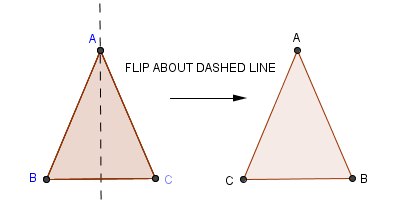﻿ Relationships Between Angles and Sides in Triangles

#Relationships Between Angles and Sides in Triangles

You may want to review:

In a triangle, we can often use information about the lengths of sides to gain information about the measures of angles. Or, we can use information about the measures of angles to gain information about the lengths of sides.

This section explores notation and results that relate the angles and sides in triangles.

First, recall the biconditional statement: to prove a sentence of the form $\,A\Longleftrightarrow B\,,$ one can prove both the forward direction $\,A\Rightarrow B\,$ and the reverse direction $\,A\Leftarrow B\,$ (that is, $\,B\Rightarrow A\,$). Together, this proves the equivalence.

The justification is the truth table below, which shows that $\,A\Longleftrightarrow B\,$  is equivalent to  $(A\Rightarrow B) \text{ and } (B\Rightarrow A)\,.$ Notice that the last two columns are identical!

 $A$ $B$ $A\Rightarrow B$ $B\Rightarrow A$ $(A\Rightarrow B)$ and $(B\Rightarrow A)$ $A\Longleftrightarrow B$ T T T T T T T F F T F F F T T F F F F F T T T T

The biconditional statement is used below to prove our first result relating sides and angles: if two sides in a triangle have the same length, then the opposite angles have the same measure. And, if two angles in a triangle have the same measure, then the opposite sides have the same lengths.

Notice what a beautiful application of SAS and ASA congruence the proof is. It's a simple and clever idea—showing that a triangle is congruent to a ‘flipped’ copy of itself.

 GIVEN: $AB=AC$ PROVE: $m\angle B=m\angle C$PROOF #1: STATEMENTS REASONS 1.  $\,AB=AC\,$ given 2.  $\,AC=AB\,$ given 3.  $\,m\angle A=m\angle A\,$ reflexive property (equality is an equivalence relation on the set of real numbers) 4. $\,\Delta ABC\cong \Delta ACB\,$ SAS 5.  $\,m\angle B=m\angle C\,$ CPCTC
 GIVEN: $m\angle B=m\angle C$ PROVE: $AB=AC$PROOF #2: STATEMENTS REASONS 1.  $\,m\angle B=m\angle C\,$ given 2.  $\,m\angle C=m\angle B\,$ given 3.  $\,BC=CB\,$ reflexive property (equality is an equivalence relation on the set of real numbers) 4.  $\,\Delta ABC\cong \Delta ACB\,$ ASA 5.   $\,AB=AC\,$ CPCTC

Together, we have:

THEOREM

Two sides of a triangle have equal lengths
$\ \Longleftrightarrow \$
the angles opposite them have equal measures.

### Proof

‘$\,\Rightarrow \,$’  See Proof #1 above.
‘$\,\Leftarrow \,$’  See Proof #2 above.
Q.E.D.

Note: ‘Q.E.D’ is an abbreviation for the Latin phrase ‘quod erat demonstrandum’ which means ‘that which was to be demonstrated (proved).’ It is often used to mark the end of a proof.

DEFINITIONS classifying triangles according to lengths of sides

A triangle is equilateral  if and only if  all its sides are equal.

A triangle is isosceles  if and only if  it has at least two equal sides.

A triangle is scalene  if and only if  all its sides have different lengths.

DEFINITIONS classifying triangles according to sizes of angles

A triangle is equiangular  if and only if  all its angles are equal.

A triangle is obtuse  if and only if  it has an obtuse angle.

A triangle is acute  if and only if  all its angles are acute.

Recall that since the angles in a triangle sum to $\,180^{\circ}\,,$ a triangle can have at most one obtuse angle.

The name theorem in mathematics is usually reserved for important results. Things that don't seem quite worthy of being called ‘theorems’ are often given other names. In particular, a corollary is usually an interesting consequence of a theorem.

Here's a corollary to the previous theorem:

COROLLARY

Every equilateral triangle is equiangular.

Every equiangular triangle is equilateral.

The proof is left to the reader.

Finally, while we're on the subject of angles and sides in a triangle, here's an interesting and useful theorem:

THEOREM
In a scalene triangle:
• the longest side is opposite the biggest angle
• the medium side is opposite the medium angle
• the shortest side is opposite the smallest angle